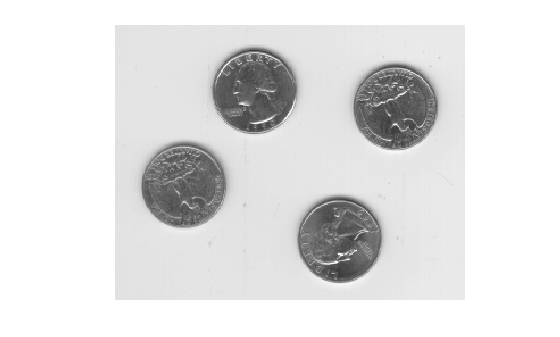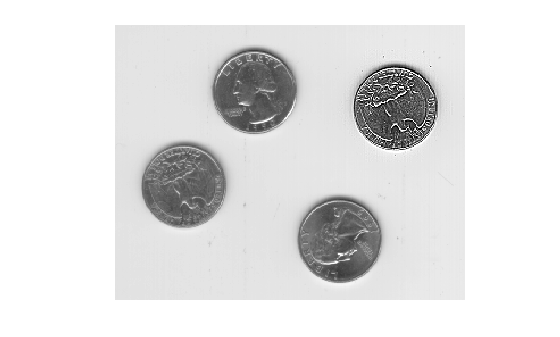# roifilt2

Filter region of interest (ROI) in image

## Syntax

``J = roifilt2(h,I,BW)``
``J = roifilt2(I,BW,fun)``

## Description

example

````J = roifilt2(h,I,BW)` filters regions of interest (ROIs) in the 2-D image `I` using the 2-D linear filter `h`. `BW` is a binary mask, the same size as `I`, that defines the ROIs in `I`. `roifilt2` returns an image that consists of filtered values for pixels in locations where `BW` contains `1`s, and unfiltered values for pixels in locations where `BW` contains `0`s.```
````J = roifilt2(I,BW,fun)` processes the data in ROIs of `I` using the function `fun`. The value `fun` must be a function handle.```

## Examples

collapse all

Read an image into the workspace.

`I = imread('eight.tif');`

Define the vertices of the mask polygon.

```c = [222 272 300 270 221 194]; r = [21 21 75 121 121 75];```

`BW = roipoly(I,c,r);`

Filter the region of the image `I` specified by the mask `BW`.

```H = fspecial('unsharp'); J = roifilt2(H,I,BW);```

Display the original image and the filtered image.

`imshow(I)````figure imshow(J)```## Input Arguments

collapse all

Linear filter, specified as a 2-D numeric matrix.

Data Types: `double`

Image, specified as a 2-D numeric matrix.

• If you specify a filter, `h`, then `I` can be any of the listed data types.

• If you specify a function handle, `fun`, then `I` can be any class supported by `fun`.

Data Types: `single` | `double` | `int8` | `int16` | `int32` | `uint8` | `uint16` | `uint32` | `logical`

Mask, specified as a 2-D logical matrix or a 2-D numeric matrix, the same size as `I`, containing `0`s and `1`s.

Data Types: `logical`

Function handle, specified as a handle. For more information about function handles, see Create Function Handle (MATLAB).

## Output Arguments

collapse all

Filtered image, returned as a 2-D matrix.

• If you specify a filter, `h`, then `J` has the same class as the input image, `I`.

• If you specify a function handle, `fun`, then the class of `J` is determined by `fun`.

## Algorithms

If you specify a filter, `h`, then `roifilt2` calls `filter2` to implement the filter.